# Anti-parallel straight lines

(diff) ← Older revision | Latest revision (diff) | Newer revision → (diff)

with respect to two given lines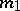and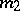Two straight lines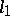and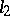which intersectandso that(cf. Fig.).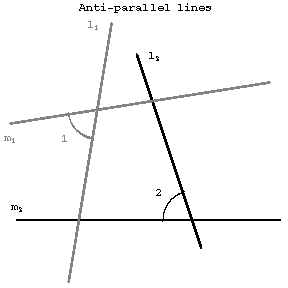Figure: a012660a

Ifandare anti-parallel with respect toand, thenandare also anti-parallel with respect toand. In any quadrilateral inscribed in a circle, any two opposite sides are anti-parallel with respect to the other two sides. If the linesandintersect at a point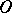, one also says thatandare anti-parallel with respect to the angle. If the linesandcoincide,andare said to be anti-parallel with respect to a straight line.

How to Cite This Entry:
Anti-parallel straight lines. Encyclopedia of Mathematics. URL: http://encyclopediaofmath.org/index.php?title=Anti-parallel_straight_lines&oldid=13704
This article was adapted from an original article by A.B. Ivanov (originator), which appeared in Encyclopedia of Mathematics - ISBN 1402006098. See original article